• 期望属于概率数学。 统计数学 1）平均值，标准差，方差 统计学里最基本的概念就是样本的均值、方差、标准差。首先，我们给定一个含有n个样本的集合，下面给出这些概念的公式描述： 均值： 方差： 标准差： ...
1、写在前面
平均值，标准差，方差，协方差都属于统计数学；期望属于概率数学。
统计数学
1）平均值，标准差，方差
统计学里最基本的概念就是样本的均值、方差、标准差。首先，我们给定一个含有n个样本的集合，下面给出这些概念的公式描述：
均值：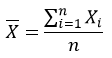方差：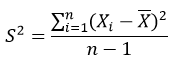标准差：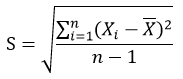均值描述的是样本集合的中间点，它告诉我们的信息是有限的。
方差（variance)是在概率论和统计方差衡量随机变量或一组数据时离散程度的度量。概率论中方差用来度量随机变量和其数学期望（即均值）之间的偏离程度。统计中的方差（样本方差）是各个数据分别与其平均数之差的平方的和的平均数。在许多实际问题中，研究方差即偏离程度有着重要意义。方差是衡量源数据和期望值相差的度量值。
而标准差给我们描述的是样本集合的各个样本点到均值的距离之平均。
以这两个集合为例，[0, 8, 12, 20]和[8, 9, 11, 12]，两个集合的均值都是10，但显然两个集合的差别是很大的，计算两者的标准差，前者是8.3后者是1.8，显然后者较为集中，故其标准差小一些，标准差描述的就是这种“散布度”。之所以除以n-1而不是n，是因为这样能使我们以较小的样本集更好地逼近总体的标准差，即统计上所谓的“无偏估计”。而方差则仅仅是标准差的平方。
方差和标准差的区别：
方差与我们要处理的数据的量纲是不一致的，多了个平方，虽然能很好的描述数据与均值的偏离程度，但是处理结果是不符合我们的直观思维的。 而标准差的根号就抵消了这个平方，就能相对直观了描述数据与均值之间的偏离程度。
2）协方差
标准差和方差一般是用来描述一维数据的，但现实生活中我们常常会遇到含有多维数据的数据集，最简单的是大家上学时免不了要统计多个学科的考试成绩。面对这样的数据集，我们当然可以按照每一维独立的计算其方差，但是通常我们还想了解更多，比如，一个男孩子的猥琐程度跟他受女孩子的欢迎程度是否存在一些联系。协方差就是这样一种用来度量两个随机变量关系的统计量，我们可以仿照方差的定义：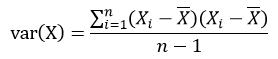来度量各个维度偏离其均值的程度，协方差可以这样来定义：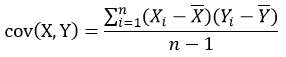协方差的结果有什么意义呢？如果结果为正值，则说明两者是正相关的（从协方差可以引出“相关系数”的定义），也就是说一个人越猥琐越受女孩欢迎。如果结果为负值， 就说明两者是负相关，越猥琐女孩子越讨厌。如果为0，则两者之间没有关系，猥琐不猥琐和女孩子喜不喜欢之间没有关联，就是统计上说的“相互独立”。
从协方差的定义上我们也可以看出一些显而易见的性质，如：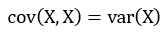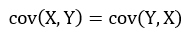3）协方差矩阵
前面提到的猥琐和受欢迎的问题是典型的二维问题，而协方差也只能处理二维问题，那维数多了自然就需要计算多个协方差，比如n维的数据集就需要计算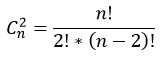个协方差，那自然而然我们会想到使用矩阵来组织这些数据。给出协方差矩阵的定义：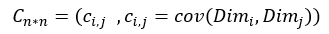这个定义还是很容易理解的，我们可以举一个三维的例子，假设数据集有三个维度，则协方差矩阵为：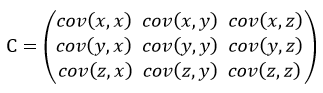可见，协方差矩阵是一个对称的矩阵，而且对角线是各个维度的方差。
概率论
1）期望（相当于统计数学中的均值）
离散型
离散型随机变量X的取值为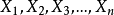，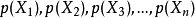为X对应取值的概率，可理解为数据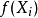出现的频率，则：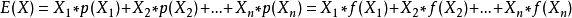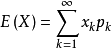连续型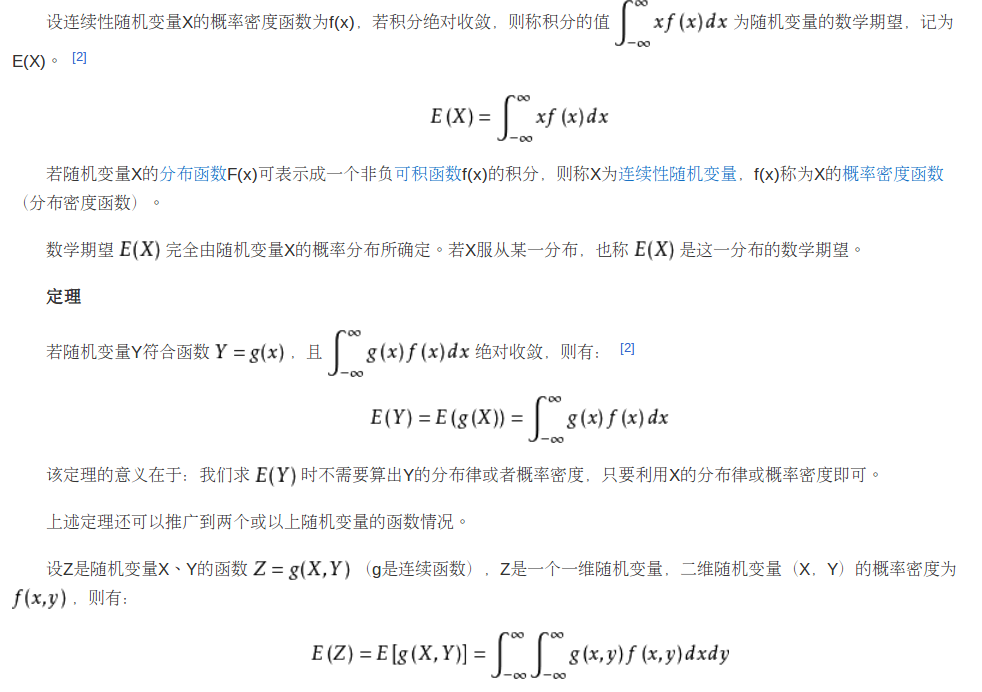2）方差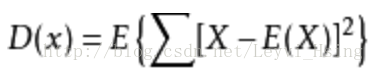E(X)表示期望，X表示原始数据，其结果就为方差。当方差很小时，X的值形成的簇比较接近它们的期望值。方差的平方根被称为标准差(standard deviation)。D(X)还可以简化为：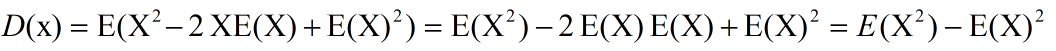这里我是这么理解的：E的作用就是求平均，既然求完平均了，那么E(X)不就是一个常数了嘛，既然是常数了，拿平均自己那还是自己呀，也就是E(E(X))那不就是E(X)嘛。既然是这样那就好理解了，E(2XE(X))=2E(X)E(X)，E(X)的平方那也是常数，求平均还是自己。
另外再看一个例子：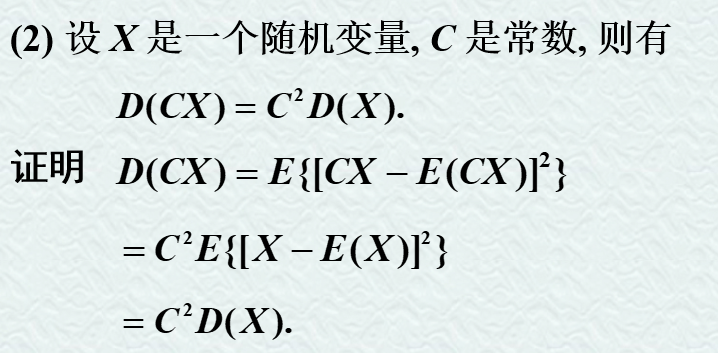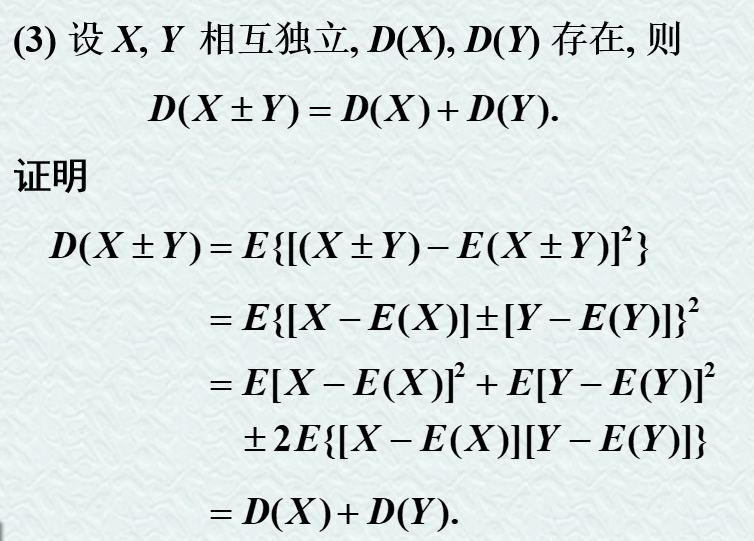3）标准差
方差的平方根被称为标准差(standard deviation)。简单来说，标准差是一组数值自平均值分散开来的程度的一种测量观念。一个较大的标准差，代表大部分的数值和其平均值之间差异较大；一个较小的标准差，代表这些数值较接近平均值。
这里标准差和方差的区别与统计学中一样。
均方误差
均方误差一般被用在机器学习的预测值与真实值之间的距离。
标准差（Standard Deviation），中文环境中又常称均方差，但不同于均方误差（mean squared error，均方误差是各数据偏离真实值的距离平方和的平均数，也即误差平方和的平均数，计算公式形式上接近方差，它的开方叫均方根误差，均方根误差才和标准差形式上接近），标准差是离均差平方和平均后的方根，用σ表示。标准差是方差的算术平方根。  从上面定义我们可以得到以下几点：  1、均方差就是标准差，标准差就是均方差  2、均方误差不同于均方差  3、均方误差是各数据偏离真实值的距离平方和的平均数  举个例子：我们要测量房间里的温度，很遗憾我们的温度计精度不高，所以就需要测量5次，得到一组数据[x1,x2,x3,x4,x5],假设温度的真实值是x，数据与真实值的误差e=x-xi  那么均方误差MSE=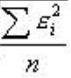总的来说，均方差是数据序列与均值的关系，而均方误差是数据序列与真实值之间的关系，所以我们只需要搞清楚真实值和均值之间的关系就行了。
展开全文• 均方误差：是各数据偏离真实值 差值的平方 的平均数；反映估计量与被估计量之间差异程度的一种度量 区别：方差是平均值，均方误差是真实值。 转换关系： 解释： 均方误差由点估计的方差与偏差的平方...
在学习回归问题评价指标时，一会遇到 方差 一会遇到均方误差（Mean Squared Error，MSE），感觉这两长得很像，所以总是搞混，所以进行一下对比：
公式：
方差：
、
均方误差：
、
概念：
方差：各数据偏离平均值（期望） 差值的平方和 的平均数
均方误差：是各数据偏离真实值    差值的平方和 的平均数；反映估计量与被估计量之间差异程度的一种度量
区别：方差是平均值，均方误差是真实值。

转换关系：

解释：
均方误差 由点估计的方差  与偏差  的平方两部分组成。
如果  是θ的无偏估计，则 ，此时用均方误差评价点估计与用方差是完全一致的，这也说明了用方差考察无偏估计是合理的。
当 不是θ的无偏估计，就要看其均方误差 ，即不仅看方差大小，还要看其偏差大小。

https://zhuanlan.zhihu.com/p/83410946
如有误，请指正，感谢！
展开全文统计学 数据分析
• ## 均方误差

万次阅读 2018-09-24 17:27:55
均方误差 一、均方误差的含义及公式 均方误差是衡量“平均误差”的一种较方便的方法，可以评价数据的变化程度。从类别来看属于预测评价与预测组合；从字面上看来，“均”指的是平均，即求其平均值，“方差”即是在...
均方误差
一、均方误差的含义及公式
均方误差是衡量“平均误差”的一种较方便的方法，可以评价数据的变化程度。从类别来看属于预测评价与预测组合；从字面上看来，“均”指的是平均，即求其平均值，“方差”即是在概率论中用来衡量随机变量和其估计值（其平均值）之间的偏离程度的度量值，“误”可以理解为测定值与真实值之间的误差。 均方误差的公式：MSE =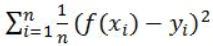。
二、均方误差的推导
均方误差可以通过平均误差来推导： 平方误差：表示实验误差大小的偏差平方和。在相同的条件下，各次测定值xi对真实值x的偏差平方后再求和，即：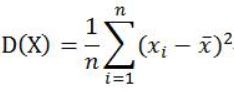均方误差是各数据偏离真实值的距离平方和的平均数，所以用真实值减去其的误差的估计值平方和求其平均数，即：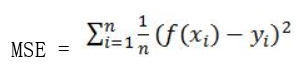估计值就是通过对之前的各个条件的x值和y值拟合出一个公式，通过公式，将新得到的x值带入求出一个y的估计值，与此同时会有一个y的真实值，再将y的估计值和真实值带入均方误差的公式中，求出一个均方误差的值，如果均方误差的值越小则说明模型的拟合实验数据能力强，但不能使它等于0，当其为0的时候则说明了这个模型完全拟合我们列出的条件，但可能现实中有我们没有考虑到的部分， 可能会使模型出现大波动，所以当均方误差值为0时，这个模型是不适用的，我们最好不要使用这个模型。
三、均方误差和均方根误差以及平均绝对误差之间的区别
为了更好的了解均方误差，我们必须要对均方误差相近的知识了解清楚，那么我们今天就来了解一下均方误差(MSE)和均方根误差(RMSE)和平均绝对误差(MAE)分别是什么： （1）均方误差（Mean Squared Error）: 均方误差是反映估计量与被估计量之间差异程度的一种度量。设t是根据子样确定的总体参数θ的一个估计量，(θ-t)2的数学期望，称为估计量t的均方误差。它等于σ2+b2，其中σ2与b分别是t的方差与偏倚。均方误差是指参数估计值与参数真值之差平方的期望值; 它可以评价数据的变化程度，当MSE的值越小时也就说明预测模型描述实验数据具有更好的精确度。 （2）均方根误差（Root Mean Square Error）：均方根误差亦称标准误差，其定义为 ，i=1，2，3，…n。在有限测量次数中，均方根误差常用下式表示：√[∑di2/n]=Re，式中：n为测量次数；di为一组测量值与真值的偏差。在有限测量次数中，均方根误差常用下式表示：√[∑di2/n]=Re，式中:n为测量次数；di为一组测量值与真值的偏差。如果误差统计分布是正态分布，那么随机误差落在±σ以内的概率为68%。均方根误差是均方误差的算术平方根。 （3）平均绝对误差（Mean Absolute Error）：又叫平均绝对离差，是所有单个观测值与算术平均值的偏差的绝对值的平均。平均绝对误差可以避免误差相互抵消的问题，因而可以准确反映实际预测误差的大小。平均绝对误差是绝对误差的平均值，平均绝对误差能更好地反映预测值误差的实际情况。
四、总结
通过这次对均方误差的学习，我们了解到了什么是均方误差、均方误差的推导是如何实现的、以及均方误差、均方根误差和平均绝对误差的区别，我们知道了如何运用均方误差来衡量一个模型是否能够较强的拟合实验数据，在以后的实践中，我们争取运用均方误差来衡量我们构建的模型是否能够实现我们想要达到的预期效果。
如果有误，望各位大神指正！
展开全文• 机器学习均方误差by ... 机器学习：均方误差和回归线简介 (Machine learning: an introduction to mean squared error and regression lines) 介绍 (Introduction) This article will deal with the statistical ...

机器学习均方误差
by Moshe Binieli
由Moshe Binieli
机器学习：均方误差和回归线简介 (Machine learning: an introduction to mean squared error and regression lines)
介绍 (Introduction)
This article will deal with the statistical method mean squared error, and I’ll describe the relationship of this method to the regression line.
本文将讨论统计方法的均方误差 ，并描述该方法与回归线的关系。
The example consists of points on the Cartesian axis. We will define a mathematical function that will give us the straight line that passes best between all points on the Cartesian axis.
该示例由笛卡尔轴上的点组成。 我们将定义一个数学函数，该函数将为我们提供在笛卡尔轴上所有点之间最佳通过的直线。
And in this way, we will learn the connection between these two methods, and how the result of their connection looks together.
这样，我们将学习这两种方法之间的联系，以及它们的联系结果如何看待。
一般说明 (General explanation)
This is the definition from Wikipedia:
这是维基百科的定义：

In statistics, the mean squared error (MSE) of an estimator (of a procedure for estimating an unobserved quantity) measures the average of the squares of the errors — that is, the average squared difference between the estimated values and what is estimated. MSE is a risk function, corresponding to the expected value of the squared error loss. The fact that MSE is almost always strictly positive (and not zero) is because of randomness or because the estimator does not account for information that could produce a more accurate estimate.

在统计中，估计器(用于估计未观察到的数量的过程的估计器)的均方误差(MSE)测量误差平方的平均值，即，估计值与估计值之间的均方差。 MSE是一个风险函数，对应于平方误差损失的期望值。 MSE几乎始终严格为正数(而不是零)的事实是由于随机性，或者是因为估算器没有考虑可能产生更准确估算的信息。

文章的结构 (The structure of the article)
Get a feel for the idea, graph visualization, mean squared error equation. 了解一下这个想法，图形可视化，均方误差方程。 The mathematical part which contains algebraic manipulations and a derivative of two-variable functions for finding a minimum. This section is for those who want to understand how we get the mathematical formulas later, you can skip it if that doesn’t interest you. 数学部分，其中包含代数操作和用于寻找最小值的二变量函数的导数。 本节适用于那些想了解我们以后如何获得数学公式的人，如果您不感兴趣，可以跳过它。 An explanation of the mathematical formulae we received and the role of each variable in the formula. 对我们收到的数学公式的解释，以及每个变量在公式中的作用。 Examples 例子
感受一下这个主意 (Get a feel for the idea)
Let’s say we have seven points, and our goal is to find a line that minimizes the squared distances to these different points.
假设我们有七个点，我们的目标是找到一条线，以最小化到这些不同点的平方距离。
Let’s try to understand that.
让我们尝试了解一下。
I will take an example and I will draw a line between the points. Of course, my drawing isn’t the best, but it’s just for demonstration purposes.
我将举一个例子，并在两点之间画一条线。 当然，我的绘画不是最好的，但这只是出于演示目的。
You might be asking yourself, what is this graph?
您可能会问自己，这张图是什么？
the purple dots are the points on the graph. Each point has an x-coordinate and a y-coordinate. 紫色点是图形上的点。 每个点都有一个x坐标和一个y坐标。 The blue line is our prediction line. This is a line that passes through all the points and fits them in the best way. This line contains the predicted points. 蓝线是我们的预测线。 这是一条穿过所有点并以最佳方式拟合它们的线。 该行包含预测点。 The red line between each purple point and the prediction line are the errors. Each error is the distance from the point to its predicted point. 每个紫色点和预测线之间的红线是错误。 每个误差是从点到其预测点的距离。
You should remember this equation from your school days, y=Mx+B, where M is the slope of the line and B is y-intercept of the line.
您应该记得上学时的方程， y = Mx + B ，其中M是直线的斜率 ， B是直线的y截距 。
We want to find M (slope) and B (y-intercept) that minimizes the squared error!
我们想要找到最小化平方误差的M( 斜率 )和B( y截距 )！
Let’s define a mathematical equation that will give us the mean squared error for all our points.
让我们定义一个数学方程式，它将为我们提供所有点的均方误差。
Let’s analyze what this equation actually means.
让我们分析一下这个方程的实际含义。
In mathematics, the character that looks like weird E is called summation (Greek sigma). It is the sum of a sequence of numbers, from i=1 to n. Let’s imagine this like an array of points, where we go through all the points, from the first (i=1) to the last (i=n). 在数学中，看起来像奇怪的E的字符称为求和(Greek sigma)。 它是从i = 1到n的数字序列的总和。 让我们想象这就像一个点数组，我们从头一个点(i = 1)到最后一个点(i = n)遍历所有点。 For each point, we take the y-coordinate of the point, and the y’-coordinate. The y-coordinate is our purple dot. The y’ point sits on the line we created. We subtract the y-coordinate value from the y’-coordinate value, and calculate the square of the result. 对于每个点，我们采用该点的y坐标和y'坐标。 y坐标是我们的紫色圆点。 y'点位于我们创建的直线上。 我们从y'坐标值中减去y坐标值，然后计算结果的平方。 The third part is to take the sum of all the (y-y’)² values, and divide it by n, which will give the mean. 第三部分是取所有(y-y')²值的总和，然后除以n，得到平均值。
Our goal is to minimize this mean, which will provide us with the best line that goes through all the points.
我们的目标是尽量减少这种均值，这将为我们提供贯穿所有要点的最佳路线。
从概念到数学方程 (From concept to mathematical equations)
This part is for people who want to understand how we got to the mathematical equations. You can skip to the next part if you want.
这部分适用于那些想了解我们如何掌握数学方程的人们 。 您可以根据需要跳到下一部分。
As you know, the line equation is y=mx+b, where m is the slope and b is the y-intercept.
如您所知，线方程为y = mx + b，其中m为斜率 ，b为y截距 。
Let’s take each point on the graph, and we’ll do our calculation (y-y’)².But what is y’, and how do we calculate it? We do not have it as part of the data.
让我们以图形上的每个点为例，进行计算(y-y')²。但是y'是什么，如何计算呢？ 我们没有将其作为数据的一部分。
But we do know that, in order to calculate y’, we need to use our line equation, y=mx+b, and put the x in the equation.
但是我们确实知道，为了计算y'，我们需要使用线方程y = mx + b，并将x放入方程中。
From here we get the following equation:
从这里我们得到以下等式：
Let’s rewrite this expression to simplify it.
让我们重写此表达式以简化它。
Let’s begin by opening all the brackets in the equation. I colored the difference between the equations to make it easier to understand.
首先打开方程式中的所有方括号。 我为方程式之间的差异着色，以使其更易于理解。
Now, let’s apply another manipulation. We will take each part and put it together. We will take all the y, and (-2ymx) and etc, and we will put them all side-by-side.
现在，让我们应用另一种操作。 我们将把各个部分放到一起。 我们将取所有y和(-2ymx)等，并将它们并排放置。
At this point we’re starting to be messy, so let’s take the mean of all squared values for y, xy, x, x².
在这一点上，我们开始变得混乱，因此让我们取y，xy，x，x²的所有平方值的平均值。
Let’s define, for each one, a new character which will represent the mean of all the squared values.
让我们为每一个定义一个新字符，它将代表所有平方值的均值。
Let’s see an example, let’s take all the y values, and divide them by n since it’s the mean, and call it y(HeadLine).
If we multiply both sides of the equation by n we get:
如果将方程式的两边都乘以n，我们将得到：
Which will lead us to the following equation:
这将导致我们得出以下等式：
If we look at what we got, we can see that we have a 3D surface. It looks like a glass, which rises sharply upwards.
如果我们看看所得到的，我们可以看到我们具有3D表面。 它看起来像玻璃，它急剧上升。
We want to find M and B that minimize the function. We will make a partial derivative with respect to M and a partial derivative with respect to B.
我们想要找到使功能最小化的M和B。 我们将对M进行偏导数，对B进行偏导数。
Since we are looking for a minimum point, we will take the partial derivatives and compare to 0.
由于我们正在寻找一个最小值，因此我们将偏导数与0进行比较。
Let’s take the two equations we received, isolating the variable b from both, and then subtracting the upper equation from the bottom equation.
让我们以接收到的两个方程为例，将变量b与两个方程隔离开，然后从底部方程中减去上部方程。
Let’s subtract the first equation from the second equation
让我们从第二个方程式中减去第一个方程式
Let’s get rid of the denominators from the equation.
让我们摆脱方程式的分母。
And there we go, this is the equation to find M, let’s take this and write down B equation.
接下来，我们找到M的方程式，让我们写下B方程式。
斜率和y截距方程 (Equations for slope and y-intercept)
Let’s provide the mathematical equations that will help us find the required slope and y-intercept.
让我们提供数学方程式，以帮助我们找到所需的斜率和y截距 。
So you probably thinking to yourself, what the heck are those weird equations?
因此，您可能在想自己，这些怪异的方程式到底有什么用？
They are actually simple to understand, so let’s talk about them a little bit.
它们实际上很容易理解，所以让我们谈谈它们。
Now that we understand our equations it’s time to get all things together and show some examples.
现在我们已经了解了方程式，现在该将所有内容放在一起并展示一些示例了。
例子 (Examples)
A big thank you to Khan Academy for the examples.
例子1 (Example 1)
Let’s take 3 points, (1,2), (2,1), (4,3).
让我们取3分，(1,2)，(2,1)，(4,3)。
Let’s find M and B for the equation y=mx+b.
让我们为方程y = mx + b找到M和B。
After we’ve calculated the relevant parts for our M equation and B equation, let’s put those values inside the equations and get the slope and y-intercept.
在为M方程和B方程计算了相关部分之后，让我们将这些值放入方程中并获得斜率和y截距 。
Let’s take those results and set them inside the line equation y=mx+b.
让我们将这些结果放在线方程y = mx + b中。
Now let’s draw the line and see how the line passes through the lines in such a way that it minimizes the squared distances.
现在，让我们画一条线，看看线如何以最小化平方距离的方式穿过线。
例子2 (Example 2)
Let’s take 4 points, (-2,-3), (-1,-1), (1,2), (4,3).
让我们取4分，(-2，-3)，(-1，-1)，(1,2)，(4,3)。
Let’s find M and B for the equation y=mx+b.
让我们为方程y = mx + b找到M和B。
Same as before, let’s put those values inside our equations to find M and B.
与以前一样，让我们​​将这些值放入方程式中以找到M和B。
Let’s take those results and set them inside line equation y=mx+b.
让我们将这些结果放在线方程y = mx + b中。
Now let’s draw the line and see how the line passes through the lines in such a way that it minimizes the squared distances.
现在，让我们画一条线，看看线如何以最小化平方距离的方式穿过线。
结论 (In conclusion)
As you can see, the whole idea is simple. We just need to understand the main parts and how we work with them.
如您所见，整个想法很简单。 我们只需要了解主要部分以及我们如何与他们合作。
You can work with the formulas to find the line on another graph, and perform a simple calculation and get the results for the slope and y-intercept.
您可以使用公式来查找另一张图上的线，并执行简单的计算并获得斜率和y截距的结果 。
That’s all, simple eh? ?
就这样，简单吗？ ？
Every comment and all feedback is welcome — if it’s necessary, I will fix the article.
欢迎提出任何意见和所有反馈-如有必要，我将修复本文。

翻译自: https://www.freecodecamp.org/news/machine-learning-mean-squared-error-regression-line-c7dde9a26b93/

机器学习均方误差

展开全文python 机器学习 人工智能 数据分析 java
• 均方误差是指参数估计值与参数真值之差平方的期望值; MSE可以评价数据的变化程度，MSE的值越小，说明预测模型描述实验数据具有更好的精确度。 RMSE 均方误差:均方根误差是均方误差的算术平方根 ...
• 方差、协方差、标准差(标准偏差/均方差）、均方误差、均方根误差(标准误差)、均方根值 本文由博主经过查阅网上资料整理总结后编写，如存在错误或不恰当之处请留言以便更正，内容仅供大家参考学习。 方差...
• ## 均方误差(MSE)

万次阅读 多人点赞 2019-08-18 13:51:30
均方误差单独扽概念是很简单的，这里只做介绍，更深一步的内容会在后面列出来。 SSE(方差、误差平方)：The sum of ...数理统计中均方误差是指参数估计值与参数真值之差平方的期望值，记为MSE。MSE是衡量“...
• 均方误差是指参数的估计值与参数的真实值之差的平方的期望. MSE可以评价数据的变化程度，MSE越小，说明模型的拟合实验数据能力强. (72)MSE=1N∑t=1N(predictedt−label)2" role="presentation" ...
• 在Excel表中，有时需要计算方差，然后根据此图绘制图表，...均方误差的概念也称为标准偏差，它是每个数据与平均值的距离的平均值. 它是平方的平方根与平均值的偏差的平均值. 标准偏差可以反映数据集的分散程度. 如...
• ## 最小均方误差

万次阅读 2017-07-26 20:41:12
最小均方误差(LMS)算法简单易行，故在系统识别、噪声去除以及信道估计等方面已得到广泛的应用。 在图像处理方面，最小均方误差法通过计算数字半调图像与原始图像在人眼视觉中的均方误差，并通过算法使其最小来获得...
• 均方误差是指参数估计值与参数真值之差平方的期望值; MSE可以评价数据的变化程度，MSE的值越小，说明预测模型描述实验数据具有更好的精确度。 RMSE 均方误差:均方根误差是均方误差的算术平方根 MAE :...
• 所以，方差、标准差是数学上的概念，而均方误差是在机器学习中用的比较多的概念，计算loss的时候会用，实际上原理是类似的，但是具体计算上稍微有些差别。这是我的理解（不一定正确），下面贴上一些具体的解释。 二...机器学习 数据分析
• 在神经网络中，如何判断一个输出向量和期望的向量有多接近呢？交叉熵（cross entropy）是常用的方法之一，刻画了两个概率分布之间的距离，是分类问题中使用较多的一种损失函数。 给定两个概率分布pq，通过q来表示...神经网络 MSE 损失函数
• MAE：Mean absolute error是绝对误差的平均值，能更好的反映预测值误差的实际情况 其中，f_i表示预测值，g_i表示真实值 缺点：若本身真实值就比较大，比如真实值1万，预测值9000，但mae=1000就会造成MAE是比较大...
• 文章目录来源方差方差与标准差均方差、均方误差、均方根误差均方根值 来源 https://blog.csdn.net/zengxiantao1994/article/details/77855644 方差 方差(variance)： 概率论中方差用来度量随机变量其数学期望（即...
• 文章目录期望（Expected value）意义定义离散型连续型期望与平均值的区别方差（Variance)案例概率论方差统计学方差样本方差python实现代码标准差（Standard Deviation)方差标准差的区别python实现代码协方差...标准差 python
• 比如对于一个4分类问题，期望输出应该为 g0=[0,1,0,0] ，实际输出为 g1=[0.2,0.4,0.4,0] ，计算g1与g0之间的差异所使用的方法，就是损失函数，分类问题中常用损失函数是交叉熵。 交叉熵（cross entropy）描述的是两...
• 均方误差——MSE 参数估计值与参数真值之差平方的期望值; 均方根误差——RMSE 平均绝对误差——MAE 绝对误差的平均值 标准差 ——SD MSE 机器学习 预测时 用，是看看预测的准不准。 SD 是看它与平均值之间的差距...
• 数理统计中均方误差是指参数估计值与参数值之差平方的期望值，记为MSE。MSE是衡量“平均误差”的一种较方便的方法，MSE可以评价数据的变化程度，MSE的值越小，说明预测模型描述实验数据具有更好的精确度。SSE（......